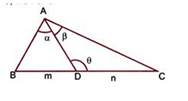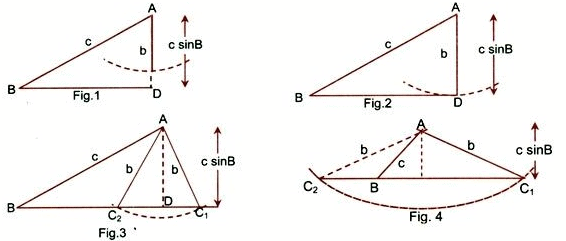Courses

# Notes | EduRev

## JEE Main Mock Test Series 2020 & Previous Year Papers

Created by: Learners Habitat

## JEE : Notes | EduRev

The document Notes | EduRev is a part of the JEE Course JEE Main Mock Test Series 2020 & Previous Year Papers.
All you need of JEE at this link: JEE
• Sine rule: Sides of a triangle are proportional to the sine of the angles opposite to them. So, in ΔABC,
sin A/a = sin B/b = sin C/c = 2Δ/abc.
This may also be written as (a/sin A) = (b/sin B) = (c/sin C)
• Cosine rule: In any ΔABC,
cos A = (b2 + c2 – a2) /2bc
cos B = (a2 + c2 – b2)/2ac
cos C = (a2 + b2 - c2)/2ab
• Trigonometric ratios of half-angles:
sin A/2 = √[(s-b)(s-c)/bc]
sin B/2 = √[(s-c) (s-a)/ac]
sin C/2 = √[(s-a) (s-b)/ab]
cos A/2 = √s(s - a)/bc
cos B/2 = √s(s - b)/ac
cos C/2 = √s(s - c)/ab
tan A/2 = √[(s - b) (s - c)/s(s - a)]
tan B/2 = √[(s - c) (s - a)/s(s - b)]
tan C/2 = √[(s - a) (s - b)/s(s - c)]
• Projection rule: In any ΔABC,
a = b cos C + c cos B
b = c cos A + a cos C
c = a cos B + b cos A
• Area of a triangle
If Δ denotes the area of the triangle ABC, then it can be calculated in any of the following forms:
Δ = 1/2 bc sin A = 1/2 ca sin B = 1/2 ab sin C
Δ = √s(s - a)(s – b)(s - c)
Δ = 1/2. (a2 sin B sin C)/ sin(B + C)
= 1/2. (b2 sin C sin A)/ sin (C + A)
= 1/2. (c2 sin A sin B)/ sin (A + B)
• Semi-perimeter of the triangle
If S denotes the perimeter of the triangle ABC, then s = (a + b + c)/2
• Napier’s analogy
In any ΔABC,
tan [(B – C)/2] = (b – c)/(b + c) cot A/2
tan [(C – A) /2] = (c – a)/(c + a) cot B/2
tan [(A – B) /2] = (a – b)/(a + b) cot C/2
• m-n theorem
Consider a triangle ABC where D is a point on side BC such that it divides the side BC in the ratio m: n, then as shown in the figure, the following results hold good:(m + n) cot θ = m cot α – n cot ß.
(m + n) cot θ = n cot B – m cot C.
• Apollonius theorem
In a triangle ABC, if AD is the median through A, then
AB+ AC2 = 2(AD2 + BD2).
• If the three sides say a, b and c of a triangle are given, then angle A is obtained with the help of the formula
tan A/2 = √(s - b) (s - c) / s(s - a) or cos A = b2 + c2 - a2 / 2bc.
Angles B and C can also be obtained in the same way.
• If two sides b and c and the included angle A are given, then
tan (B – C) /2 = (b – c)/ (b + c) cot A/2
This gives the value of (B- C)/2.
Hence, using (B + C)/2 = 90o - A/2 along with the last equation both B and C can be evaluated. Now, the sides can be evaluated using the formula
a = b sin A/sin B or a2 = b2 + c2 – 2bc cosA.
• If two sides b and c and the angle B (opposite to side b) are given, then using the following results, we can easily obtain the remaining elements
sin C = c/b sinB, A = 180– (B + C) and b = b sin A/sinB
• Some of the important points which must be remembered include:
1. b < c sin B, there is no triangle possible (Fig. 1)
2. If b = c sin B and B is an acute angle, then only one triangle is possible (Fig. 2)
3. If c sin B < b < c and B is an acute angle, then there are two values of angle C (Fig. 3).
4. If c < b and B is an acute angle, then there is only one triangle (Fig. 4).Offer running on EduRev: Apply code STAYHOME200 to get INR 200 off on our premium plan EduRev Infinity!

## JEE Main Mock Test Series 2020 & Previous Year Papers

3 videos|174 docs|149 tests

,

,

,

,

,

,

,

,

,

,

,

,

,

,

,

,

,

,

,

,

,

;